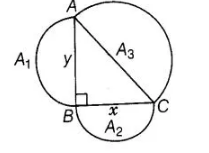# Prove that the area of the semi-circle drawn

Question:

Prove that the area of the semi-circle drawn on the hypotenuse of a right angled triangle is equal to the sum of the areas of the semi-circles drawn on

the other two sides of the triangle.

Solution:

Let ABC be a right triangle, right angled at B and AB = y, BC = x.

Three semi-circles are drawn on the sides AB, BC and AC, respectively with diameters AB, BC and AC, respectively.

Again, let area of circles with diameters AB, BC and AC are respectively A1, A2 and A3.To prove $A_{3}=A_{1}+A_{2}$

Proof $\ln \triangle A B C$, by Pythagoras theorem,

$A C^{2}=A B^{2}+B C^{2}$

$\Rightarrow$ $A C^{2}=y^{2}+x^{2}$

$\Rightarrow$ $A C=\sqrt{y^{2}+x^{2}}$

We know that, area of a semi-circle with radius, $r=\frac{\pi r^{2}}{2}$

$\therefore$ Area of semi-circle drawn on $A C, A_{3}=\frac{\pi}{2}\left(\frac{A C}{2}\right)^{2}=\frac{\pi}{2}\left(\frac{\sqrt{y^{2}+x^{2}}}{2}\right)^{2}$

$\Rightarrow$ $A_{3}=\frac{\pi\left(y^{2}+x^{2}\right)}{8}$ ...(i)

Now, area of semi-circle drawn on $A B, A_{1}=\frac{\pi}{2}\left(\frac{A B}{2}\right)^{2}$

$\Rightarrow$ $A_{1}=\frac{\pi}{2}\left(\frac{y}{2}\right)^{2} \Rightarrow A_{1}=\frac{\pi y^{2}}{8}$ ...(ii)

and area of semi-circle drawn on $B C, A_{2}=\frac{\pi}{2}\left(\frac{B C}{2}\right)^{2}=\frac{\pi}{2}\left(\frac{x}{2}\right)^{2}$

$\Rightarrow$ $A_{2}=\frac{\pi x^{2}}{8}$

On adding Eqs. (ii) and (iii), we get $A_{1}+A_{2}=\frac{\pi y^{2}}{8}+\frac{\pi x^{2}}{8}$

$=\frac{\pi\left(y^{2}+x^{2}\right)}{8}=A_{3} \quad$ [from Eq. (i)]

$\Rightarrow \quad A_{1}+A_{2}=A_{3}$ Hence proved.